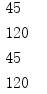• #include<iostream> using namespace std; int f(int a) { int b = 0; static int c = 3; a = c++,b++; return (a); } int main() { int a = 2, i, k; for (i = 0; i < 2; i++) ... ret
#include<iostream>
using namespace std;

int f(int a)
{
int b = 0;
static int c = 3;
a = c++,b++;
return (a);
}
int main()
{
int a = 2, i, k;
for (i = 0; i < 2; i++)
k = f(a++);
printf(" % d\n", k);

return 0;
}


答案：4 解析：因为逗号表达式的优先级最低，所有先计算赋值操作符，再计算逗号操作符，
展开全文• ## 语句执行频度

千次阅读 2019-06-10 16:43:34
语句执行频率分别是N、N(N-1)/2、N(N-1)(N-2)/6 ①1+2+3+...+n-1 ②1+2+3+...n-2 ... 前n项和为N*(N-1)*(N-2)/6   证明： 1+2+3+……+N=N(N+1)/2=(N²+N)/2 1²+2²+……N²=N(N+1)(2N+1)/6 ...
最近看算法4中遇到的以前没注意到的问题：
求数组中三个数和为0的个数ThreeSum
package com.learn.mstake;

/**
* @Author: shixiaofei
* @Description:
* @Date: 2019/6/10 16:20
* @Version: 1.0
*/

public class ThreeSum {

public static void main(String[] args) {
int arr[] = new int[]{1, -1, 0, 2, -2, 0, 1, 2, 3};
int count = count(arr);
System.out.println(count);
}

public static int count(int[] a) {
int length = a.length;
int cnt = 0;
for (int i = 0; i < length; i++) {
for (int j = i + 1; j < length; j++) {
for (int k = j + 1; k < length; k++) {
if (a[i] + a[j] + a[k] == 0) cnt++;
}
}
}
return cnt;
}
}

语句执行频率分别是N、N(N-1)/2、N(N-1)(N-2)/6
①1+2+3+...+n-1
②1+2+3+...n-2
...
前n项和为N*(N-1)*(N-2)/6

证明：
1+2+3+……+N=N(N+1)/2=(N²+N)/2 1²+2²+……N²=N(N+1)(2N+1)/6 所以数列各项加起来就是： S(N)=(1²+1)/2+(2²+2)/2+(3²+3)/2+……+(N²+N)/2 =[(1²+2²+3²+……+N²)+(1+2+3+……+N)]/2 =[N(N+1)(2N+1)/6+N(N+1)/2]/2 =N(N+1)[(2N+1)/6+1/2]/2 =N(N+1)(N+2)/6
其中n=n-2
附：
1²+2²+...+n²
(n+1)^3 - n^3 = 3*n^2 + 3*n + 1，可以得到下列等式： 2^3 - 1^3 = 3*1^2 + 3*1 + 1 3^3 - 2^3 = 3*2^2 + 3*2 + 1 4^3 - 3^3 = 3*3^2 + 3*3 + 1 ......... (n+1)^3 - n^3 = 3.n^2 + 3*n + 1 以上式子相加得到 (n+1)^3 - 1 = 3*Sn + 3*n(n+1)/2 + n 其中Sn = 1^2 + 2^2 + 3^2 + ...... + n^2 化简整理得到： Sn = n*(n + 1)*(2n + 1)/6
 static int count;
static int count1;
public static void main(String[] args) {
int n=10;
anInt(n);
System.out.println(count1);
System.out.println(count);
int sum1=n*(n-1)/2;
int sum3=(n-2)*(n-1)*n/6;
System.out.println(sum1);
System.out.println(sum3);
}

public static void anInt(int n) {

for (int i = 0; i < n; i++) {
for (int j = i + 1; j < n; j++) {
count1++;
for (int k = j + 1; k < n; k++) {
count++;
}
}
}
}
输出结果：展开全文• 以下代码执行后输出结果为（ ） public class Test { public static void main(String[] args) { System.out.println("return value of getValue(): " + getValue()); } public static int getValu...
以下代码执行后输出结果为（ ）
public class Test {
public static void main(String[] args) {
System.out.println("return value of getValue(): " +
getValue());
}
public static int getValue() {
try {
return 0;
} finally {
return 1;
}
}
}
A.return value of getValue(): 1
B.return value of getValue(): 0
C.return value of getValue(): 0return value of getValue(): 1
D.return value of getValue(): 1return value of getValue(): 0

本题选A。
根据官方的JVM规范：如果try语句里有return，返回的是try语句块中变量值。 详细执行过程如下：
如果有返回值，就把返回值保存到局部变量中；执行jsr指令跳到finally语句里执行；执行完finally语句后，返回之前保存在局部变量表里的值。
如果try，finally语句里均有return，忽略try的return，而使用finally的return.

展开全文• 执行下列程序是输入，123<空格>456<空格>789<回车>,输出的结果是？ main() {char s; int c,i; scanf("%c",&c); scanf("%d",&i); scanf("%s",s); printf("%c,%d,%s\n",c,i,s); }解释一下，谢谢你们printf
• 在一个技术QQ群中,试着做了一道C的题...原题： 在C语言中，下列代码的输出结果是 （ ） (选择一项) void main( ) {  int i = 0, j = 10;  for(i=0;ij;i++,j--)  {  if(j==10)  {  printf("%d
 在一个技术QQ群中,试着做了一道C的题，感觉是考察for循环的执行流程.
原题： 在C语言中，下列代码的输出结果是 （ ） (选择一项)

void
main( ) {
int
i
=

0
, j
=

10
;
for
(i
=
0
;i
<
j;i
++
,j
--
)     {
if
(j
==
10
)         {             printf(
"
%d,%d
"
,i,j);             j
--
;         }         j
++
;     }     printf(
"
%d,%d
"
,i,j); }    a)
0
,
10

9
,
9
b)
0
,
10

10
,
10
c)
10
,
10
d)
9
,
11

群里居然有不少人都选了B，那个提问者还一直在问正确的结果。
最终的正确结果是：A.
哈哈，好基本的知识。
后来偶就顺手写了一个代码，大家做了它可以对for循环的运算顺序就比较清楚啦。

int
print1(
int
i,
int
j) {             printf(
"
执行判断条件,i=%d,j=%d
"
,i,j);
return

1
; }
void
main( ) {
int
i
=

0
, j
=

10
;
for
(i
=
0
;((print1(i,j))
&&
(i
<
j));         i
++
,j
--
,printf(
"
执行for()中的第三个语句,i=%d,j=%d
"
,i,j))     {
if
(j
==
10
)         {             printf(
"
%d,%d
"
,i,j);             j
--
;         }         j
++
;         printf(
"
执行for循环体中的最后一句,i=%d,j=%d
"
,i,j);     }     printf(
"
%d,%d
"
,i,j); }

运行结果是：
执行判断条件,i=0,j=10 0,10
执行for循环体中的最后一句,i=0,j=10 执行for()中的第三个语句,i=1,j=9
执行判断条件,i=1,j=9 执行for循环体中的最后一句,i=1,j=10 执行for()中的第三个语句,i=2,j=9
执行判断条件,i=2,j=9 执行for循环体中的最后一句,i=2,j=10 执行for()中的第三个语句,i=3,j=9
执行判断条件,i=3,j=9 执行for循环体中的最后一句,i=3,j=10 执行for()中的第三个语句,i=4,j=9
执行判断条件,i=4,j=9 执行for循环体中的最后一句,i=4,j=10 执行for()中的第三个语句,i=5,j=9
执行判断条件,i=5,j=9 执行for循环体中的最后一句,i=5,j=10 执行for()中的第三个语句,i=6,j=9
执行判断条件,i=6,j=9 执行for循环体中的最后一句,i=6,j=10 执行for()中的第三个语句,i=7,j=9
执行判断条件,i=7,j=9 执行for循环体中的最后一句,i=7,j=10 执行for()中的第三个语句,i=8,j=9
执行判断条件,i=8,j=9 执行for循环体中的最后一句,i=8,j=10 执行for()中的第三个语句,i=9,j=9
执行判断条件,i=9,j=9
9,9 --------------
结论：
for(expression1;expresson2;expression3)
{
expression4;
}
先执行expression1,判断expresson2是否成立，若成立，则执行expression4;
再执行expression3;判断expression2是否成立，若成立，则再执行expression4;
再执行expression3;判断expression2是否成立，若成立，则再执行expression4;
.......
直到expression2不成立，则退出循环。
展开全文• explain显示了MySQL如何使用索引来处理select语句以及...这个不重要,查询序号即为sql语句执行的顺序 select_type select类型，它有以下几种值  simple 它表示简单的select,没有union和子查询  primary 最外面的selsql 索引 优化 select mysql
• 数据库表中字段上创建的索引，在写SQL语句进行查询时，索引可能会被使用到，也肯能因为SQL语句导致索引在此条SQL语句执行时索引无效。 聚簇索引的顺序就是数据的物理存储顺序，而对非聚簇索引的解释是:索引顺序与...数据库索引 索引
• 无需在执行存储过程和触发器的每个语句后向客户端发送 DONE_IN_PROC 消息。 29.尽量避免大事务操作，提高系统并发能力。 30.尽量避免向客户端返回大数据量，若数据量过大，应该考虑相应需求是否合理 1、避免...
• ## SQL语句的执行顺序

千次阅读 2013-01-23 10:38:43
sql语句执行步骤： 1）语法分析，分析语句的语法是否符合规范，衡量语句中各表达式的意义。 2） 语义分析，检查语句中涉及的所有数据库对象是否存在，且用户有相应的权限。 3）视图转换，将涉及视图的查询...
• 四、print输出语句 输出语句的语法格式：Print() #在python3中print换成函数，必须加括号。# 4.1 字符串输出： ########################################## print输出字符串的时候必须用引号引起来，否则会报...python 基础 笔记
• 6.for i in range(2,n):if n%i==0:breakif i==n-1:print('是素数')...8.对于有else子句的for循环和while循环，但循环因循环条件不成立而自然结束时__会__(会或不会)执行else中的代码。10.已知x='hello world.',那...
• 执行i++3、现在知道是先执行i++，再执行判断语句，i++不是++吗，例如i为1，那i++后执行判断语句，i是几呢？i是2.4、好像i++和++i在for（）里时结果都一样。都一样，for语句头的执行顺序决定了这两个写法都一样，...语言 c
• 前言：有java编程基础的人对java的异常处理机制都会有一定了解，而且可能感觉使用起来也比较简单，但如果在try catch finally语句块中遇到return语句，开发者可能就会遇到一些逻辑问题，甚至步入编程的陷阱。...java 异常处理 return
• 通过分析SQL语句执行计划优化SQL(总结)做DBA快7年了，中间感悟很多。在DBA的日常工作中，调整个别性能较差的SQL语句时一项富有挑战性的工作。其中的关键在于如何得到SQL语句执行计划和如何从SQL语句执行计划中...sql 优化 oracle table access
• 通过分析SQL语句执行计划优化SQL(总结) FROM:http://www.blogjava.net/shanben/archive/2008/07/07/213150.html   做DBA快7年了，中间感悟很多。在DBA的日常工作中，调整个别性能较差的SQL语句时一项富有...
• 一：下面介绍带有输出参数和返回值的存储过程的创建方法 1,创建存储过程 create proc p5 @cno char(10),@ccredit decimal (5,1) output as begin if exist (select * from course where c_no=@cno) update ...
• 题目（阿里笔试题）：下面代码在64位Linux系统编译执行输出结果是__。#include <stdint.h> #include <stdio.h> void print_size(int32_t array){ printf("%d\n", sizeof(array)); } int main () { int...
• ## switch语句

万次阅读 多人点赞 2019-02-17 19:36:16
1. 语句结构 语法形式 switch语句从字面上讲，可以称为开关语句，当然理解上不要以为就只有开和关，可以想象它是一个多路开关。它是一种多分支结构。 switch语句的语法形式为： switch(整型表达式) { case ...
• 如果你用的是 Oracle 8i 及以上的版本，那简单，在过程中用 execute immediate sql_str 就行, sql_str 是一个拼凑的 SQL 语句，但这个动态语句中带参数，或 Select 的结果要 into 到变量中时就要稍加留心一下了。...
• ## 高效SQL语句必杀技

万次阅读 多人点赞 2012-03-29 13:11:04
一是查询优化器为当前的SQL语句生成最佳的执行计划，保证数据读写使用最佳路径；二是设置合理的物理存储结构，如表的类型，字段的顺序，字段的数据类型等。本文主要描述如何编写高效的SQL语句并给出示例。下面的描述...sql statistics object access oracle
• ## C#语句_if语句

万次阅读 2020-01-24 10:27:19
if C#关于if语句的定义 不带else的if语句 带else的if语句 C#关于if语句的定义 if 语句根据布尔表达式的值选择要执行语句。 if-statement: if ( boolean-expression ) embedded-statement if ( boolean-expression ...迭代 多重循环
• 条件语句用于根据不同条件执行不同动作。 PHP条件语句 当您编写代码的时候，您常常需要为不同的判断执行不同的动作。您可以在代码当中使用条件语句来完成这个任务。 在PHP当中，提供了下列条件语句： if语句 ...
• 本文主要介绍使用Statement的execute()方法来执行任意的SQL语句，并列出你在运行该程序中可能会遇到的一系列问题，我只能将我在运行中遇到的问题描述，希望对你有所帮助。首先，你需要创建一个配置数据库的配置文件...jdbc mysql 学习笔记 mysql.ini Java
• 在实际的程序中，存在很多需要重复执行的流程，为了简化这些重复的执行流程，在程序设计语言中新增了该类语句。  在学习循环语句时，最重要的就是发现流程的规律，然后再用程序设计语言将该规律描述出来，从来实现...
• osql /U alma /P /i stores.bat (stores.bat中包含update ,delete语句,不过,不需要使用GO) ---------------------------------------------------- osql 实用工具 osql 实用工具使您得以输入 Transact-...sql sqlserver 服务器 工具 file
• ## Arduino常用语句

千次阅读 2021-01-20 20:09:56
一 控制语句 if语句 if (表达式) 语句 else 语句2 if (表达式1) 语句 else if (表达式1) 语句2 ... 语句3 ...switch语句 ...//先执行语句，判断为真继续循环 for语句 for (表达式1; 表达式2; 表达arduino...